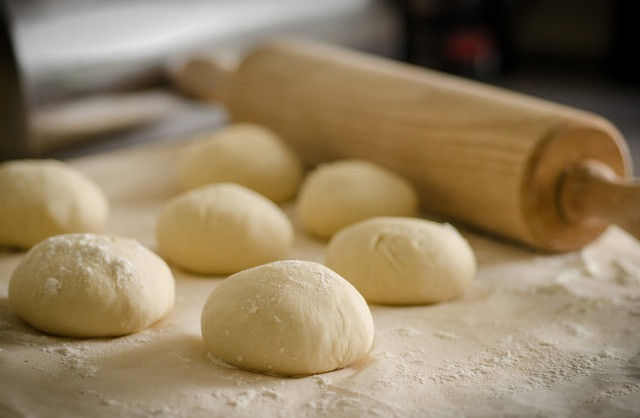# Cooking with Fractions

Student View (Opens in new window)## Instructor Overview

Students determine how many times they would need to fill a quarter cup to measure the ingredients in a recipe.

# Key Concepts

This lesson informally introduces the idea of dividing by a fraction. Students must figure out how many times a quarter cup must be filled to measure the ingredients in a recipe. This involves dividing each amount by $\frac{1}{4}$. Here are some methods students might use:

• Add $\frac{1}{4}$ repeatedly until the sum is the desired amount. Count the number of $\frac{1}{4}$s that were added.
• Start with the amount in the recipe. Subtract $\frac{1}{4}$ repeatedly until the difference is 0. Count the $\frac{1}{4}$s that were subtracted.
• Draw a model (e.g., a bar or a number line model) to represent the amount in the recipe. Divide it into fourths and count the number of fourths.

# Goals and Learning Objectives

• Learn how to divide by a fraction.